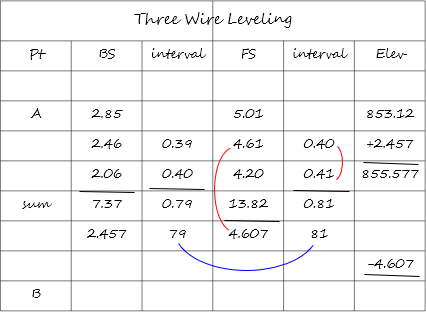## 3. Field notes

Field notes may seem complex at first, but that's only because of all the readings and running computations. Wire readings are recorded top-middle-bottom in the respective column (BS or FS). Computations are done immediately to catch reading errors and determine distances.

An example serves to illustrate how the notes are compiled.

A circuit is run from point A (elev = 853.12) to point B then to point C. The rod numbers on the diagrams are the top, middle, and bottom wire readings. The stadia multiplier for the instrument used is 100.

### a. Set up the field note left plate

The interval column will be used to perform distance computations### b. Set up midway between points A and B

#### (1) Backsight point A and record readings in BS columnFigure G-3BS on Point A#### (2) Running computationsAdd the readings and divide by three for the BS value:  2.85+2.46+2.06=7.37;     7.37/3 = 2.457

The average (2.457) should be approximately the same as the middle reading (2.46).

If it isn't, that means there is at least one reading error; check the readings again.

Add the average BS to the elevation of point A to get the elevation of the instrument: 853.12+2.457 = 855.577

Compute the sub-intervals: (top - middle) and (middle - bottom) readings; 2.85-2.46 = 0.39 and 2.46-2.06 = 0.40.

These should be approximately equal to each other, taking into account reading errors.

Add the sub-intervals to obtain the rod interval, i: 0.39+0.40 = 0.79

Apply the stadia multiplier to get the distance: H = 100. (0.79) = 79'

#### (3)  Rotate to and take FS readings on point B; Record in FS columnFigure G-4FS on Point B#### (4) Running computations

Perform the same computations for the FS readings as were done for the BS readings.Subtract the average FS reading from the instrument elevation to get the elevation of point B: 855.577-4.607 = 850.970

The FS distance should be close to the BS distance (81' and 79') in order for distance-based systematic errors to cancel.

### c. Move instrument to between points B and C.

#### (1) Backsight on point B; Record in BS columnFigure G-5BS on Point B

#### (2) Running computations#### (3) FS on point C; Record in FS columnFigure G-6FS on Point C

#### (4) Running computations### d. Perform a page check

As in differential leveling, a page check should be perform to catch math errors. Two setups will fit on a single plate leaving enough space for a page check.

The page check is: Begin elev + BS sum - FS sum = End elev